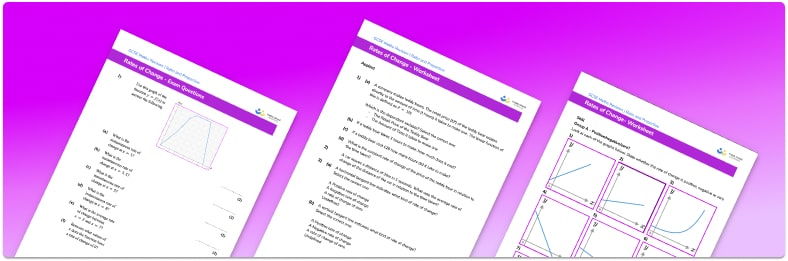# Rate Of Change Worksheet• Section 1 of the rate of change worksheet contains 18 skills-based rate of change questions, in 3 groups to support differentiation
• Section 2 contains 4 applied rate of change questions with a mix of word problems and deeper problem solving questions
• Section 3 contains 4 foundation and higher level GCSE exam style practice questions
• An answer key and a mark scheme for all
• All questions are created by fully qualified expert 400;”>exam questions are provided
• Questions follow variation theory with plenty of secondary maths teachers
• Suitable for GCSE maths revision for AQA, OCR and Edexcel exam boards

• This field is for validation purposes and should be left unchanged.

You can unsubscribe at any time (each email we send will contain an easy way to unsubscribe). To find out more about how we use your data, see our privacy policy.

### Rate of change at a glance

The rate of change of a variable is a measure of how quickly that variable changes over time. A good example of this is acceleration, which is a measure of how quickly an object’s speed changes over time.

If we draw a graph of how a variable changes over time, for example a speed-time graph, the rate of change is represented by the gradient, or slope of the line, sometimes called the unit rate. A constant rate of change is shown by a straight line and if the variable doesn’t change for a period of time, this is represented by a horizontal line, with gradient 0.

From a graph, we can calculate the average rate of change between two points by calculating the slope of a line drawn between the two points.

When the rate of change is not constant, the graph will show a curved line. We can estimate the rate of change at any given point in time by estimating the gradient of the line at that point. An example of a real life graph with a non constant rate of change is the money in a savings account with a compound interest rate. The y intercept would represent the initial value, the initial amount of money in the account, and the rate of change would increase each year.

Note that the gradient of a line between two ordered pairs (x1, y2) and (x2,y2) is found using the gradient, or slope formula: (y2-y1)/(x2-x1). The slope-intercept form of a straight line is written as y=mx+c, where m is the gradient (the rate of change) and c is the y-intercept.

Looking forward, students can then progress to additional rate of change worksheets and other ratio and proportion worksheets, for example a ratio worksheet or a simplifying and equivalent ratios worksheetFor more teaching and learning support on Ratio and Proportion our GCSE maths lessons provide step by step support for all GCSE maths concepts.

When you have students who require more intensive support our one to one GCSE maths revision programme will match them with the most appropriate tutor. This way we can provide individual students with personalised programmes of study while you continue to teach the rest of your class as a whole group.

Our maths interventions are currently only available for GCSE students, and are not suitable for A level students.

## Do you have KS4 students who need more focused attention to succeed at GCSE?There will be students in your class who require individual attention to help them succeed in their maths GCSEs. In a class of 30, it’s not always easy to provide.

Help your students feel confident with exam-style questions and the strategies they’ll need to answer them correctly with our dedicated GCSE maths revision programme.

Lessons are selected to provide support where each student needs it most, and specially-trained GCSE maths tutors adapt the pitch and pace of each lesson. This ensures a personalised revision programme that raises grades and boosts confidence.

Find out more